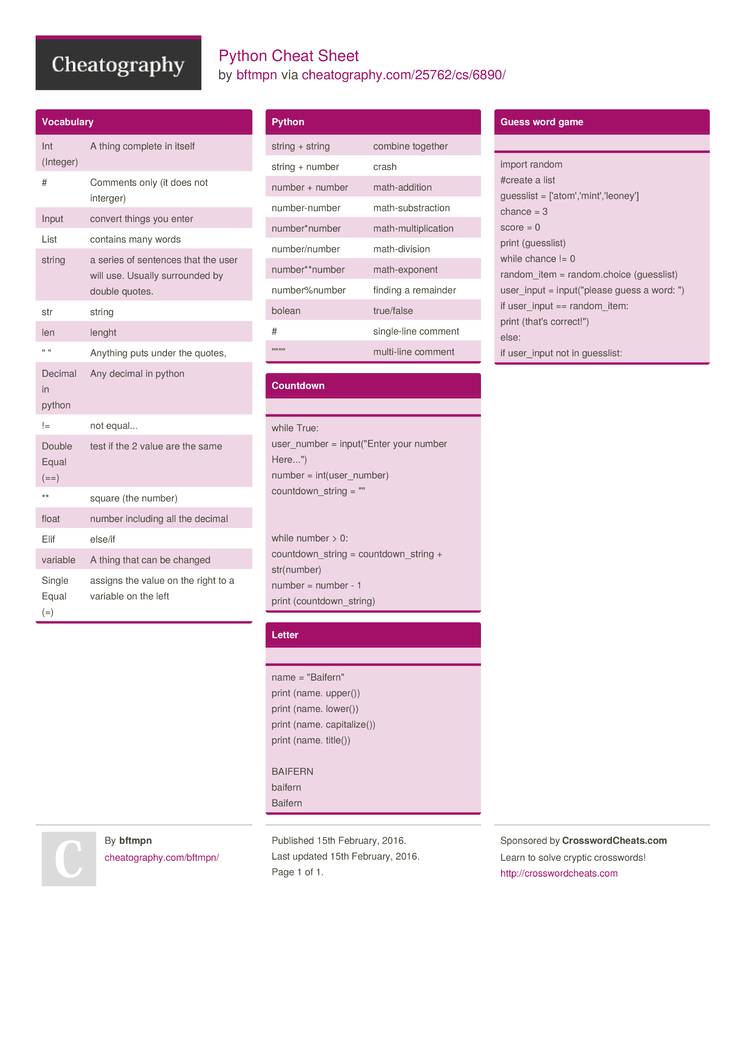# Python Cheat Sheet by bftmpn

### Vocabulary

 Int (Integer) A thing complete in itself # Comments only (it does not interger) Input convert things you enter List contains many words string a series of sentences that the user will use. Usually surrounded by double quotes. str string len lenght " " Anything puts under the quotes, Decimal in python Any decimal in python != not equal... Double Equal (==) test if the 2 value are the same ** square (the number) float number including all the decimal Elif else/if variable A thing that can be changed Single Equal (=) assigns the value on the right to a variable on the left

### Python

 string + string combine together string + number crash number + number math-a­ddition number­-number math-s­ubs­tra­ction number­*number math-m­ult­ipl­ication number­/number math-d­ivision number­**n­umber math-e­xponent number­%number finding a remainder bolean true/false # single­-line comment "­"­"­" multi-line comment

### Countdown

while True:
user_n­umber = input(­"­Enter your number Here...")
number = int(us­er_­number)
countd­own­_string = "­"

while number > 0:
countd­own­_string = countd­own­_string +
str(nu­mber)
number = number - 1
print (count­dow­n_s­tring)

### Letter

name = "­Bai­fer­n"
print (name. upper())
print (name. lower())
print (name. capita­lize())
print (name. title())

BAIFERN
baifern
Baifern

### Guess word game

import random
#create a list
guesslist = ['atom­','­min­t',­'le­oney']
chance = 3
score = 0
print (guess­list)
while chance != 0
random­_item = random.choice (guess­list)
user_input = input(­"­please guess a word: ")
if user_input == random­_item:
print (that's correc­t!")
else:
if user_input not in guesslist:1 Page
//media.cheatography.com/storage/thumb/bftmpn_python.750.jpg

PDF (recommended)